# Samacheer Kalvi Books: Tamilnadu State Board Text Books Solutions

## Samacheer Kalvi 10th Maths Chapter 4 Geometry Notes PDF Download: Tamil Nadu STD 10th Maths Chapter 4 Geometry NotesSamacheer Kalvi 10th Maths Chapter 4 Geometry Notes PDF Download: Tamil Nadu STD 10th Maths Chapter 4 Geometry Notes

We bring to you specially curated Samacheer Kalvi 10th Maths Chapter 4 Geometry Notes PDF which have been prepared by our subject experts after carefully following the trend of the exam in the last few years. The notes will not only serve for revision purposes, but also will have several cuts and easy methods to go about a difficult problem.

 Board Tamilnadu Board Study Material Notes Class Samacheer Kalvi 10th Maths Subject 10th Maths Chapter Chapter 4 Geometry Format PDF Provider Samacheer Kalvi Books

## How to Download Samacheer Kalvi 10th Maths Chapter 4 Geometry Notes PDFs?

2. Click on the Samacheer Kalvi 10th Maths Notes PDF.
3. Look for your preferred subject.
4. Now download the Samacheer Kalvi 10th Maths Chapter 4 Geometry notes PDF.

## Tamilnadu Samacheer Kalvi 10th Maths Solutions Chapter 4 Geometry Unit Exercise 4

Question 1.
In the figure, if BD⊥AC and CE ∠ AB, prove thatSolution:
In the figure’s ∆AEC and ∆ADB.
We have ∠AEC =∠ADB = 90 (∵ CE ∠AB and BD ∠AC)
and ∠EAC =∠DAB
[Each equal to ∠A]
Therefore by AA-criterion of similarity, we have ∆AEC ~ ∆ADB
(ii) We have
∆AEC ~ ∆ADB [As proved above]
CABA=ECDBCAAB=CEDB
Hence proved.

Question 2.
In the given figure AB||CD || EF . If AB = 6 cm, CD = x cm, EF = 4 cm, BD = 5 cm and DE = y cm. Find x and y.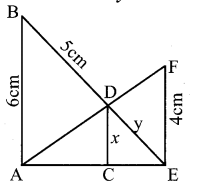Solution:
In the given figure, ∆AEF, and ∆ACD are similar ∆s.
∠AEF = ∠ACD = 90°
∠A = ∠A (common)
∴ ∆AEF ~ ∆ACD (By AA criterion of similarity)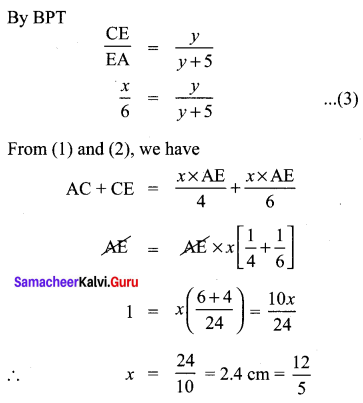Subtstituting x = 2.4 cm in (3)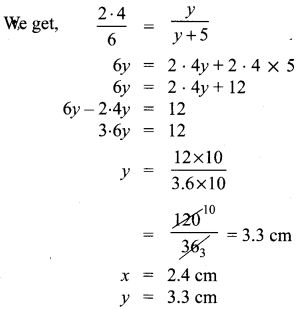Question 3.
O is any point inside a triangle ABC. The bisector of ∠AOB , ∠BOC and ∠COA meet the sides AB, BC and CA in point D, E and F respectively.
Show that AD × BE × CF = DB × EC × FA
Solution:
In ∆AOB, OD is the bisector of ∠AOB.In ∆BOC, OE is the bisector of ∠BOC
OBOC=BEEC …………. (2)
In ∆COA, OF is the bisector of ∠COA.
OCOA=CFFA …………… (3)
Multiplying the corresponding sides of (1), (2) and (3), we get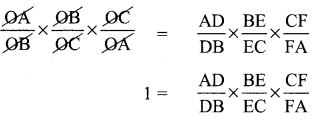⇒ DB × EC × FA = AD × BE × CF
Hence proved.

Question 4.
In the figure, ABC is a triangle in which AB = AC . Points D and E are points on the side AB and AC respectively such that AD = AE . Show that the points B, C, E and D lie on a same circle.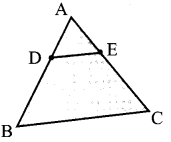Solution:
In order to prove that the points B, C, E and D are concyclic, it is sufficient to show that ∠ABC + ∠CED = 180° and ∠ACB + ∠BDE = 180°.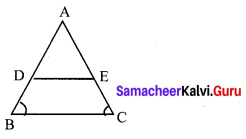In ∆ABC, we have AB = AC and AD = AE.
⇒ AB – AD = AC – AE
⇒ DB = EC
Thus we have AD = AE and DB = EC. (By the converse of Thale’s theorem)
⇒ ∠ABC + ∠BDE = ∠ADE + ∠BDE (Adding ∠BDE on both sides)
⇒ ∠ABC + ∠BDE = 180°
⇒ ∠ACB + ∠BDE = 180° (∵ AB = AC ∴ ∠ABC = ∠ACB)
Again DE || BC
⇒ ∠ACB = ∠AED
⇒ ∠ACB + ∠CED = ∠AED + ∠CED (Adding ∠CED on both sides).
⇒ ∠ACB + ∠CED = 180° and
⇒ ∠ABC + ∠CED = 180° (∵ ∠ABC = ∠ACB)
Thus BDEC is a quadrilateral such that
⇒ ∠ACB + ∠BDE = 180° and
⇒ ∠ABC + ∠CED = 180°
∴ BDEC is a cyclic quadrilateral. Hence B, C, E, and D are concyclic points.

Question 5.
Two trains leave a railway station at the same time. The first train travels due west and the second train due north. The first train travels at a speed of 20 km/hr and the second train travels at 30 km/hr. After 2 hours, what is the distance between them?
Solution:
After 2 hours, let us assume that the first train is at A and the second is at B.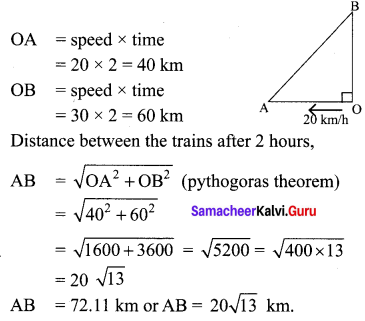Question 6.
D is the mid point of side BC and AE⊥BC. If i BC a, AC = b, AB = c, ED = x, AD = p and AE = h , prove that
(i) b2 = p2 + ax + a24
(ii) c2 = p2 – ax + a24
(iii) b2 + c2 = 2p2 + a22
Solution:
From the figure, D is the mid point of BC.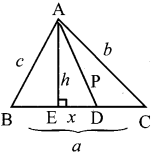We have ∠AED = 90°

AC2 = AD2 + DC2 + 2DC × DE
⇒ AC2 = AD2 + 12 BC2 + 2 . 12 BC . DE
⇒ AC2 = AD2 + 14 BC2 + BC . DE
⇒ AC2 = AD2 + BC . DE + 14 BC2
⇒ b2 = p2 + ax + 14 a2
Hence proved.

(ii) In ∆ABD, ∠ADE is an acute angle.
AB2 = AD2 + BD2 – 2BD . DE
⇒ AB2 = AD2 + (12BC)2 – 2 × 12 BC . DE
⇒ AB2 = AD2 + 14 BC2 – BC . DE
⇒ AB2 = AD2 – BC . DE + 14 BC2
⇒ c2 = p2 – ax + 14 a2
Hence proved.

(iii) From (i) and (ii) we get .
AB2 + AC2 = 2AD2 + 12 BC2
i.e. c2 + b2 = 2p2 + a22
Hence it is proved.

Question 7.
A man whose eye-level is 2 m above the ground wishes to find the height of a tree. He places a mirror horizontally on the ground 20 m from the tree and finds that if he stands at a point C which is 4 m from the mirror B, he can see the reflection of the top of the tree. How height is the tree?
Solution:
From the figure; ∆DAC, ∆FBC are similar triangles and ∆ACE & ∆ABF are similar triangles.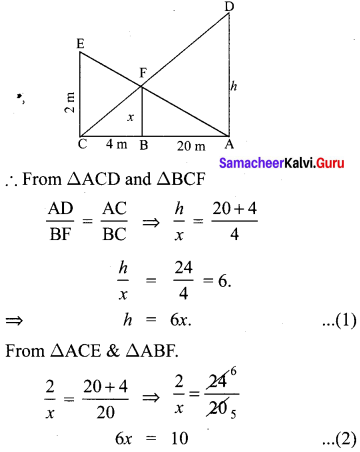∴ height of the tree h = 6x = 10 m.

Question 8.
An emu which is 8 ft tall standing at the foot of a pillar which is 30 ft height. It walks away from the pillar. The shadow of the emu falls beyond emu. What is the relation between the length of the shadow and the distance from the emu to the pillar?
Solution:
Let OA (emu shadow) the x and AB = y.
⇒ pillar’s shadow = OB = OA + AB
⇒ OB = x + y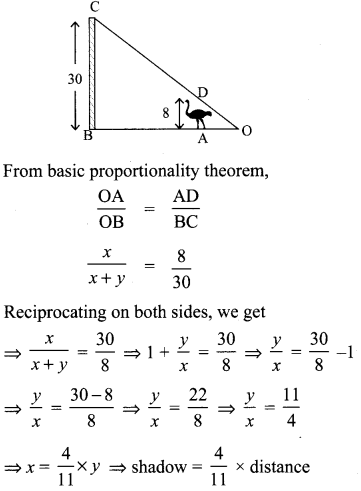Question 9.
Two circles intersect at A and B. From a point P on one of the circles lines PAC and PBD are drawn intersecting the second circle at C and D. Prove that CD is parallel to the tangent at P.
Solution:
Let XY be the tangent at P.
TPT: CD is || to XY.
Construction: Join AB.
∠BAC + ∠BDC= 180° ………… (1)
∠BDC = 180° – ∠BAC …………. (2)
Equating (1) and (2)
we get ∠BDC = ∠PAB
Similarly we get ∠PBA = ∠ACD
as XY is tangent to the circle at ‘P’
∠BPY = ∠PAB (by alternate segment there)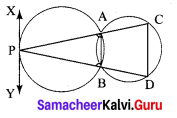∴ ∠PAB = ∠PDC
∠BPY = ∠PDC
XY is parallel of CD.
Hence proved.

Question 10.
Let ABC be a triangle and D, E, F are points on the respective sides AB, BC, AC (or their extensions). Let AD: DB = 5 : 3, BE : EC = 3 : 2 and AC = 21. Find the length of the line segment CF.
Solution: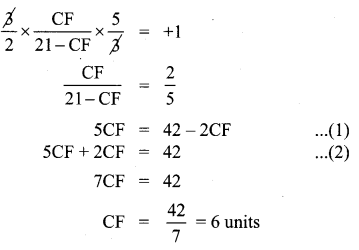## Tamilnadu Samacheer Kalvi 10th Maths Solutions Chapter 4 Geometry Additional Questions

Question 1.
In figure if PQ || RS, Prove that ∆POQ ~ ∆SOR
Solution:
PQ || RS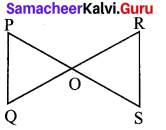So, ∠P = ∠S (A Hernate angles)
and ∠Q = ∠R
Also, ∠POQ = ∠SOR (vertically opposite angle)
∴ ∆POQ ~ ∆SOR (AAA similarity criterion)

Question 2.
In figure OA . OB = OC . OD Show that ∠A = ∠C and ∠B = ∠D
Solution:
OA . OB = OC . OD (Given)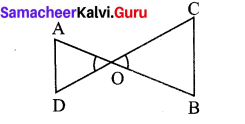Also we have ∠AOD = ∠COB
(vertically opposite angles) …………. (2)
From (1) and (2)
∴ ∆AOD ~ ∆COB (SAS similarity criterion)
So, ∠A = ∠C and ∠B = ∠D
(corresponding angles of similar triangles)

Question 3.
In figure the line segment XY is parallel to side AC of ∆ABC and it divides the triangle into two parts of equal areas. Find the ratio AXAB
Solution:
Given XY||AC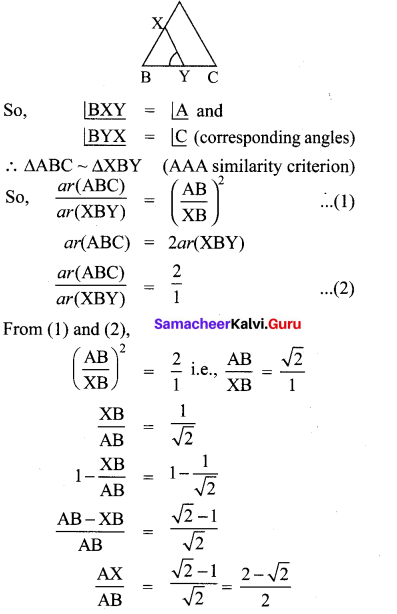Question 4.
In AD⊥BC, prove that AB2 + CD2 = BD2 + AC2.
Solution:
AC2 = AD2 + CD2 …………… (1)
(Pythagoras theorem)
AB2 = AD2 + BD2 …………. (2)
(Pythagoras theorem)
Subtracting (1) from (2) we have,
AB2 – AC2 = BD2 – CD2
AB2 + CD2 = BD2 + AC2

Question 5.
BL and CM are medians of a triangle ABC right angled at A.
Prove that 4(BL2 + CM2) = 5BC2.
Solution:
BL and CM are medians at the ∆ABC in which
A = ∠90°.
From ∆ABC
BC2 = AB2 + AC2 …………… (1)
(Pythagoras theorem)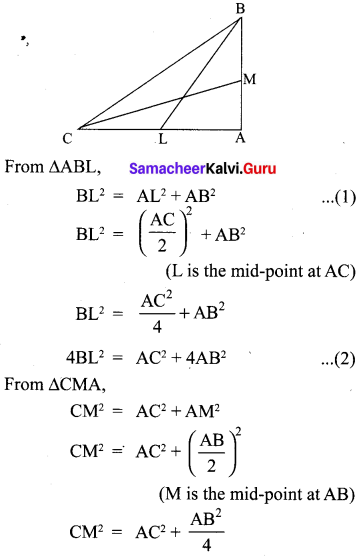4CM2 = 4AC2 + AB2 …………… (3)
Adding (2) and (3), we have
4(BL2 + CM2) = 5(AC2 + AB2)
4(BL2 + CM2) = 5BC2 [From (1)]

Question 6.
Prove that in a right triangle, the square of the hypotenure is equal to the sum of the squares of the others two sides.
Solution:
Proof: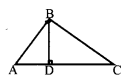We are given a right triangle ABC right angled at B.
We need to prove that AC2 = AB2 + BC2
Let us draw BD ⊥ AC
AD . AC = AB2 …………. (1)
Also, ∆BDC ~ ∆ABC
CDBC=BCAC
CD . AC = BC2 …………. (2)
AD . AC + CD . AC = AB2 + BC2
AC(AD + CD) = AB2 + BC2
AC . AC = AB2 + BC2
AC2 = AB2 + BC2

Question 7.
A ladder is placed against a wall such that its foot is at a distance of 2.5 m from the wall and its top reaches a window 6 m above the ground. Find the length of the ladder.
Solution:
Let AB be the ladder and CA be the wall with the window at A.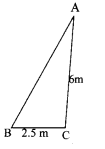Also, BC = 2.5 m and CA = 6 m
From Pythagoras theorem,
AB2 = BC2 + CA2
= (2.5)2 + (6)2
= 42.25
AB = 6.5
Thus, length at the ladder is 6.5 m.

Question 8.
In figure O is any point inside a rectangle ABCD. Prove that OB2 + OD2 = OA2 + OC2.
Solution:
Through O, draw PQ||BC so that P lies on AB and Q lies on DC.
Now, PQ||BC
PQ⊥AB and PQ⊥DC (∵ ∠B = 90° and ∠C = 90°)
So, ∠BPQ = 90° and ∠CQP = 90°
Therefore BPQC and APQD are both rectangles.
Now from ∆OPB,
OB2 = BP2 + OP2 ……………. (1)
Similarly from ∆OQD,
OD2 = OQ2 + DQ2 …………. (2)
From ∆OQC, we have
OC2 = OQ2 + CQ2 ……….. (3)
∆OAP, we have
OA2 = AP2 + OP2 …………. (4)
OB2 + OD2 = BP2 + OP2 + OQ2 + DQ2 (As BP = CQ and DQ = AP)
= CQ2 + OP2 + OQ2 + AP2
= CQ2 + OQ2 + OP2 + AP2
= OC2 + OA2 [From (3) and (4)]

Question 9.
In ∠ACD = 90° and CD⊥AB. Prove that BC2AC2=BDAD
Solution:
∆ACD ~ ∆ABC
So,Question 10.
The perpendicular from A on side BC at a ∆ABC intersects BC at D such that DB = 3 CD. Prove that 2AB2 = 2AC2 + BC2.
Solution:
We have DB = 3 CD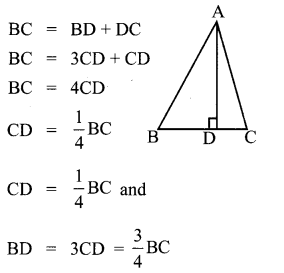Since ∆ABD is a right triangle (i) right angled at D
AB2 – AD2 + BD2 …………. (ii)
By ∆ACD is a right triangle right angled at D
AC2 = AD2 + CD2 ………… (iii)
Subtracting equation (iii) from equation (ii),
we got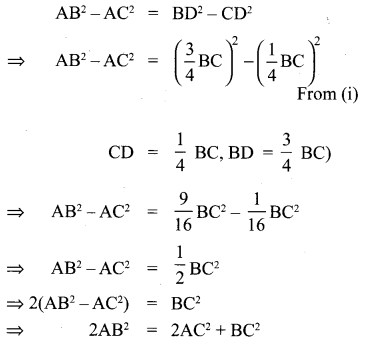## How to Prepare using Samacheer Kalvi 10th Maths Chapter 4 Geometry Notes PDF?

Students must prepare for the upcoming exams from Samacheer Kalvi 10th Maths Chapter 4 Geometry Notes PDF by following certain essential steps which are provided below.

• Use Samacheer Kalvi 10th Maths Chapter 4 Geometry notes by paying attention to facts and ideas.
• Pay attention to the important topics
• Refer TN Board books as well as the books recommended.
• Correctly follow the notes to reduce the number of questions being answered in the exam incorrectly
• Highlight and explain the concepts in details.

## Frequently Asked Questions on Samacheer Kalvi 10th Maths Chapter 4 Geometry Notes

#### How to use Samacheer Kalvi 10th Maths Chapter 4 Geometry Notes for preparation??

Read TN Board thoroughly, make separate notes for points you forget, formulae, reactions, diagrams. Highlight important points in the book itself and make use of the space provided in the margin to jot down other important points on the same topic from different sources.

#### How to make notes for Samacheer Kalvi 10th Maths Chapter 4 Geometry exam?

Read from hand-made notes prepared after understanding concepts, refrain from replicating from the textbook. Use highlighters for important points. Revise from these notes regularly and formulate your own tricks, shortcuts and mnemonics, mappings etc.
Share: